# System Identification

## Multi-model identification

Consider a simple linear stochastic system of the form

 dx = Ax dt + B dw;dy = Cx dt + du (1)

with the state and observation noise terms dw and du being independent Wiener processes. We consider the (A,B,C) triple as unknown, and the goal of this research program is to identify (A,B,C) given the observations dy(t). This may be framed as a nonlinear filtering problem, and generally such filtering problems require an infinite number of sufficient statistics. However, the assumption that the unknown variables come from a finite set leads to a finite set of sufficient statistics. It is shown in  that if the triple (A,B,C) comes from a finite set of models {(Ai,Bi,Ci) | i = 1,2,,N} and if the prior state probability is Gaussian then we may propagate the conditional probability pi(t) that the observations are generated by the ith model via: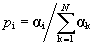with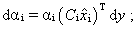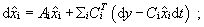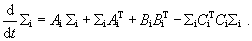(2)

Here {ai}, {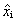}, and {Si } are the sufficient statistics.  The stochastic differential equation for ai is an Itô equation.

The assumption that the candidate model set is finite is too strong; we might, however, assume that each unknown lies within an open interval. If the true system parameters (A,B,C) = (A(q), B(q), C(q)) with q Î Rn  unknown, then we may think of the system and all models as n-vectors lying in some open ball in Rn.  Now if the true model q is not in the candidate model set, then propagating equations (2) will result in the model which most closely approximates the true model having the greatest pi .  We propose the following multi-model multi-iteration algorithm:

 1.                Choose an initial set of N candidate models. 2.                Use the observations dy([0,T1]) to propagate the {pi}s. 3.                Choose a new set of candidate models about the model with the greatest pi(T1). 4.                Repeat steps 2 and 3 ad infinitum: a.       For the jth model set, use the observations dy ([Tj-1, Tj]) to propagate the jth set of {pi}s. b.      Choose the (j+1)th set of candidate models about the model with the greatest pi(Tj)

Ideally, as t increases to infinity we would like the Euclidean distance between the true system and the models to decrease to zero. That is, lim t®¥ max k |q - qk| = 0.  The choice of T­j may depend on {pi(t)}, i.e. the use of a stopping rule. We also need to decide how to choose the candidate models. Finally, we are looking for a way of continuously adapting the model set, rather than adapting at discrete times as above.

We wish to show that the above multi-model multi-iteration algorithm converges with probability 1 to the true model. We also wish to contrast with other identification schemes, such as stochastic approximation . Does the parallel computation inherent in our algorithm increase the convergence rate to the true model?

## Nuclear Magnetic Resonance

The entire research program is motivated by physical problems involving nuclear magnetic resonances . Consider the system

 dx = [(A + Du) x + b] dt + B dw;dy = Cx dt + du. (3)

Now we want to identify the (A,B,b,C,D) quintuple as well as select a control u(t) which increases the convergence rate to the true model. There are experimental identification techniques currently used in the NMR community, and one question that we ask is how optimal are those methods, i.e. how efficient are they in using the observations dy to decrease the uncertainty in the unknown model.

## References

             R.W. Brockett, N. Khaneja, and S. Glaser. Optimal input design for NMR system identification. Proceedings of the 2001 Conference on Decision and Control.

            B.D.O. Anderson and J. Moore. Optimal Filtering. Prentice-Hall, 1979.

             T.E. Duncan and B. Pasik-Duncan. Adaptive control of continuous-time linear stochastic systems. Mathematics of Control, Signals, and Systems, 3:45-60, 1990.

             D.G. Lainiotis. Optimal adaptive estimation: structure and parameter adaptation. IEEE Transactions on Automatic Control, Vol: AC-16 (2), April 1971.